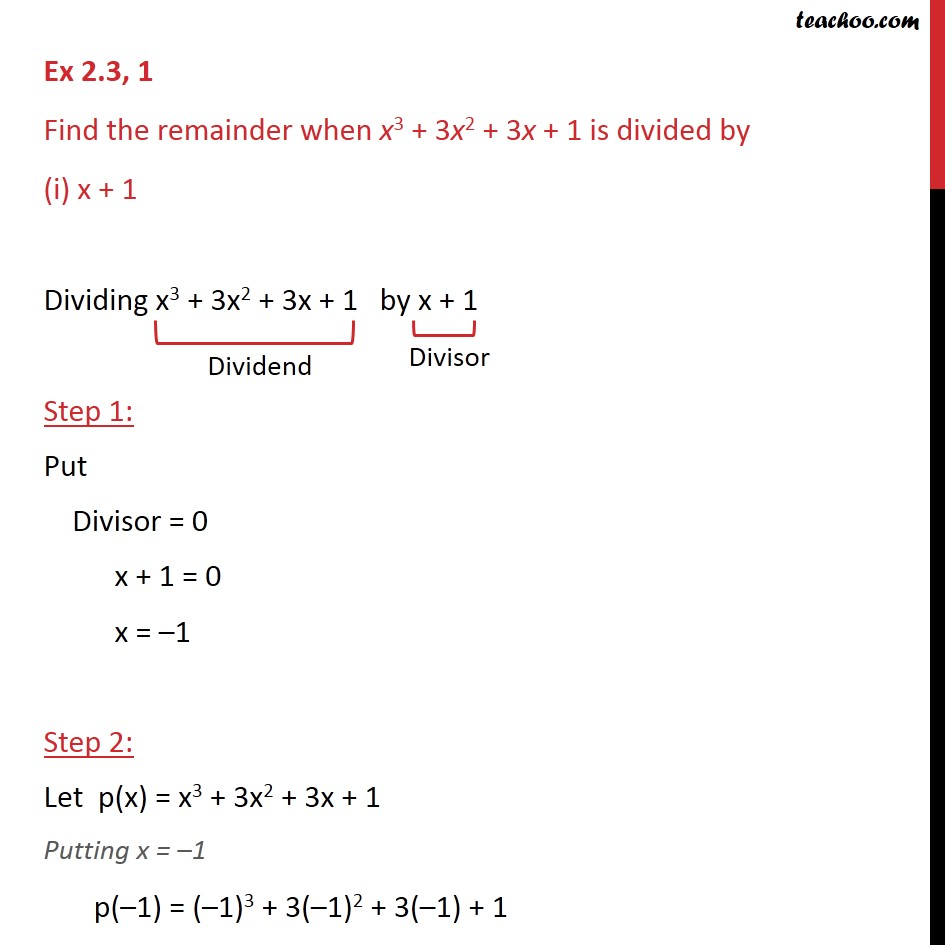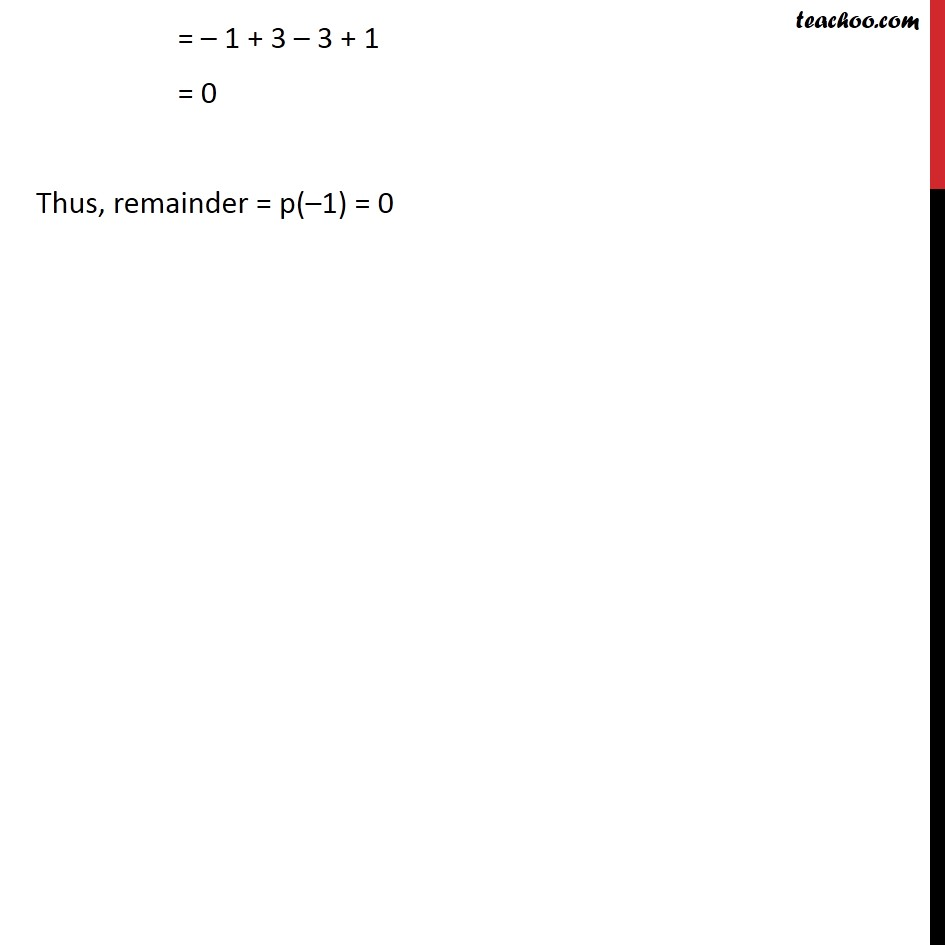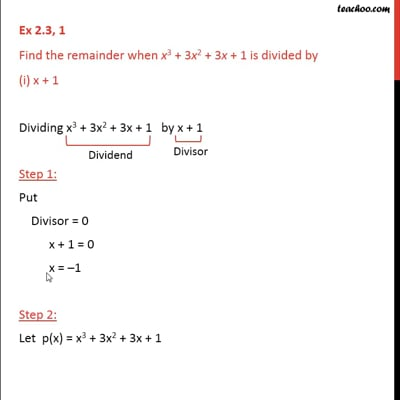Remainder Theoram

Chapter 2 Class 9 Polynomials
Concept wiseThis video is only available for Teachoo black users

Introducing your new favourite teacher - Teachoo Black, at only ₹83 per month

### Transcript

Ex 2.3, 1 Find the remainder when x3 + 3x2 + 3x + 1 is divided by (i) x + 1 Dividing x3 + 3x2 + 3x + 1 by x + 1 Step 1: Put Divisor = 0 x + 1 = 0 x = –1 Step 2: Let p(x) = x3 + 3x2 + 3x + 1 Putting x = –1 p(–1) = (–1)3 + 3(–1)2 + 3(–1) + 1 = – 1 + 3 – 3 + 1 = 0 Thus, remainder = p(–1) = 0